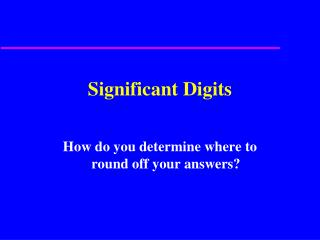DownloadDownload PresentationSignificant Digits

# Significant Digits

Download Presentation## Significant Digits

- - - - - - - - - - - - - - - - - - - - - - - - - - - E N D - - - - - - - - - - - - - - - - - - - - - - - - - - -
##### Presentation Transcript

1. Significant Digits How do you determine where to round off your answers?

2. Counting Significant Digits • 1. A nonzero digit is always significant. • 2. Zeros between nonzero digits are always significant. • 3. Zeros at the beginning of a number are never significant. • 4. Zeros at the end of a number are significant only if there is a decimal in the number. (trailing zero or ending zero)

3. Examples • 4.156 g. has 4 significant figures • 3.0067 cm. has 5 sf • .24 mm. has 2 sf • .024 cm. has 2 sf • .00024 m. also has 2 sf • 3.00 ml. has 3 sf, but • 300 ml only has 1 sf

4. a. 3.336 b. 504.7 c. .00302 d. 6.00 x 106 e. 0.0060 f. 32707 g. 3520.0 4 sf 4 sf 3 sf 3 sf 2 sf 5 sf 5 sf How many SF are in each of these numbers?

5. Adding and Subtracting • Round off the answer to the same precision as the least precise factor. • For example, 2.37 is precise to the hundredths place, but 3.3 is only precise to the tenths place. When these two numbers are added, the answer, 5.67 should be rounded off to 5.7.

6. Multiplying and Dividing • Count the number of significant digits in each factor. • Round off your answer to the same number of significant digits as the factor with the fewest significant digits.

7. Determine the Number of Significant Digits • 5.432 g • 40.319 g • 146 cm • 0.189 kg • 0.000235 g • 1.04 x 105 g • 0.04050 L

8. Perform the following function. Your answer should have the correct number of Significant Digits. • 12 cm + 5 cm • 3.5 m + 1.26 m • 7.00 g – 2.4 g • 4 cm x 3 cm • 5.0 mm x 1.25 mm • 60 pm x 110 pm • 2.45 g ÷ 5 mL